Filters: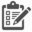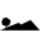××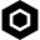×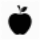××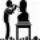×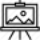×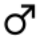×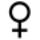××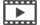××
×
×
×
×
×
×
×
×
×
×
×
×
×